• 2021-05-03 07:01:28

Ridge regression is a method for estimating coefficients of

linear models that include linearly correlated predictors.

Coefficient estimates for multiple linear regression models rely on the

independence of the model terms. When terms are correlated and the columns of the

design matrix X have an approximate linear dependence, the matrix

(XTX)–1

is close to singular. Therefore, the least-squares estimate

β^=(XTX)−1XTy

is highly sensitive to random errors in the observed response

y, producing a large variance. This situation of

multicollinearity can arise, for example, when you collect data without an

experimental design.

Ridge regression addresses the problem of multicollinearity by estimating

regression coefficients using

β^=(XTX+kI)−1XTy

where k is the ridge parameter and I is the

identity matrix. Small, positive values of k improve the

conditioning of the problem and reduce the variance of the estimates. While biased,

the reduced variance of ridge estimates often results in a smaller mean squared

error when compared to least-squares estimates.

更多相关内容
• matlab中岭回归的实现，分析financial distress数据库中的误差和MSPE，可视化
• ridge_regression 用于岭回归的python代码（已实现以预测下个月的CO2浓度） 资料可用性 文件 Ridge.py ：标准函数和Ridge回归函数window_make.py ：使用滑动窗口方法制作大小为p（窗口大小）的时间序列列表。 Final...
• 高光谱解混数据集（Japser Ridge），matlab的mat文件。原始数据有512 x 614 个像素。每个像素记录在范围从380nm到2500nm的224个通道中。光谱分辨率高达9.46nm。由于这个高光谱图像太复杂而无法得到基本事实，因此...
• 具有l2-norm（Ridge回归）的线性回归的Python实现 用法 专案追踪志上的一系列网志文章 每个算法都包含在其自己的文件夹中。 依存关系 Python 3+ 麻木 matplotlib仅用于演示 免责声明 仅用于教育目的。 使用风险...Python
• 资源来自pypi官网。 资源全名：ridge_detection-2.0.1.tar.gzPython库
• 早午餐框架 概述 首先，感谢项目Croissant，swtpm维护程序和Chromebrew框架的工作，这些工作在创建该项目时得到了积极的利用。 Brunch框架的目的是根据官方恢复映像创建通用的x86_64 ChromeOS映像。...
• 用于HPC Linux群集的shell / R程序，允许用户在混合模型框架内估算用于内核Ridge回归的最佳衰减率参数，以进行预测。 最佳衰减率是使用K倍交叉验证（使用群集节点并行化）估算的。开源软件
• Ridge-Slope-Valley功能用于指纹活动性检测研究论文
• Finger-vein based biometrics, as a new approach to personal identification, has received much attention in recent years. However, the poor visibility of finger-vein imageries is really not beneficial ...
• Local Local Path Planning Based on Ridge Regression Extreme Learning Machines for an Outdoor Robot研究论文
• Section I: Brief Introduction on Three Regression Models Regulation is one approach to tackle the problem of overfitting by adding additional information, and thereby shrinking the parameter values of...
• 介绍了Linear ， Ridge和Kernel Ridge回归的相关约束。 以它们的标准形式，我们可以将这些模型公式化为形式的无约束优化问题 minimize L(y, yhat) 其中y是目标值，而yhat是预测值。通过添加相关约束并得出相关约束...
• 岭回归代码加注释详解，附longley数据集------Ridge Regression回归
• 参考 6.2.2 Kernel Ridge Regression, An Introduction to Support Vector Machines and other Kernel-based Learning Methods, Nello Cristianini 和 John Shawe-Taylor 参考 7.3.2 Kernel Methods for Pattern ...matlab
• 如果惩罚项是参数的l_2范数，就是岭回归(Ridge Regression)；如果惩罚项是参数的l_1范数，就是套索回归（Lasso Regrission）。本篇从几何和代数两个角度解释为什么Lasso更倾向于稀疏解，Ridge更倾向于稠密解，

为了限制模型参数的数值大小，就在模型原来的目标函数上加上一个惩罚项，这个过程叫做正则化（Regularization）。

• 如果惩罚项是参数的 l 2 l_2 范数，就是岭回归(Ridge Regression)
• 如果惩罚项是参数的 l 1 l_1 范数，就是套索回归（Lasso Regrission）

Ridge是在结构风险最小化的正则化因子上使用模型参数向量的二阶范数形式，Lasso使用的是一阶范数形式。虽然Ridge可以将参数估计值向0进行收缩，但对于任何调优参数值，它都不能将系数取值变为严格的0。尽管某些参数估计值变得非常小以至于可以忽略，但事实上Ridge并没有进行变量选择。这可能对预测精确度来说不是问题，但却对模型解释提出了挑战，尤其在变量个数大的时候。一种流行的用来替代Ridge的模型是“最小绝对收缩与选择算子”模型，通常被称为LassoLasso不仅将参数估计向0收缩，当调优参数足够大时，一些参数估计将直接缩减为零，这可以达到特征选择的作用。这样一来，Lasso回归的结果更易于解释。

可能有人会问从RidgeLasso，只是罚函数从二阶范数变成一阶范数，为什么Lasso就能够将参数估计收缩成0而Ridge不能呢？要回答这个问题，我们先看下LassoRidge分别对应的另一版本的等价优化方程。对于Lasso而言，优化下面两个方程是等价的：

Σ i = 1 n ( y i − β 0 − Σ j = 1 p β j x i j ) 2 + λ Σ j = 1 p ∣ β j ∣ = R S S + λ Σ j = 1 p ∣ β j ∣ m i n β { Σ i = 1 n ( y i − β 0 − Σ j = 1 p β j x i j ) 2 } ,   Σ j = 1 p ∣ β j ∣ ≤ s (1) \Sigma_{i=1}^{n}(y_{i}-\beta_{0}-\Sigma_{j=1}^{p}\beta_{j}x_{ij})^{2}+\lambda\Sigma_{j=1}^{p}|\beta_{j}|=RSS+\lambda\Sigma_{j=1}^{p}|\beta_{j}|\\ \underset{\beta}{min}\left\{ \Sigma_{i=1}^{n}\left(y_{i}-\beta_{0}-\Sigma_{j=1}^{p}\beta_{j}x_{ij}\right)^{2}\right\} ,\ \Sigma_{j=1}^{p}|\beta_{j}|\leq s\tag{1}

也就是说，对每个调优参数 λ \lambda 的取值，都存在相应的 s s 值，使得上面两个方程优化后得到的参数估计相同。类似的，对于Ridge，下面两个方程等价：

Σ i = 1 n ( y i − β 0 − Σ j = 1 p β j x i j ) 2 + λ Σ j = 1 p β j 2 = R S S + λ Σ j = 1 p β j 2 m i n β { Σ i = 1 n ( y i − β 0 − Σ j = 1 p β j x i j ) 2 } ,   Σ j = 1 p β j 2 ≤ s (2) \Sigma_{i=1}^{n}(y_{i}-\beta_{0}-\Sigma_{j=1}^{p}\beta_{j}x_{ij})^{2}+\lambda\Sigma_{j=1}^{p}\beta_{j}^{2}=RSS+\lambda\Sigma_{j=1}^{p}\beta_{j}^{2}\\ \underset{\beta}{min}\left\{ \Sigma_{i=1}^{n}\left(y_{i}-\beta_{0}-\Sigma_{j=1}^{p}\beta_{j}x_{ij}\right)^{2}\right\} ,\ \Sigma_{j=1}^{p}\beta_{j}^{2}\leq s\tag{2}

当 p ＝ 2 p＝2 时，Lasso的参数估计是所有满足 ∣ β 1 ∣ + ∣ β 2 ∣ ≤ s |\beta_1|+|\beta_2|≤s β 1 \beta_1 β 2 \beta_2 取值中最小化RSS的。Ridge是估计所有满足 β 1 2 + β 2 2 ≤ s \beta_1^2+β_2^2\leq s 的参数取值中最小化RSS的。当 s s 很大时，相应的限制条件几乎是无效的，只要参数估计能够最小化RSS即使绝对值很大也没有问题。只要 s s 所定义的区域包含最小二乘解，那么收缩方法得出的参数估计和一般最小二乘回归就相同。相反，如果 s s 很小，那么可能的参数取值范围就很有限。

下面看看看看LassoRidge的不同之处左边是Lasso对应的误差等位线和正方形限制区域，右边是Ridge对应的等位线和圆形限制区域。 上面图中围绕在 β ^ \hat{\beta} 周围的椭圆表示有相同RSS的参数估计。随着椭圆的扩大，对应的RSS增加。LassoRidge的估计值就是在一定的限制区域下，椭圆不断扩张的过程中和限制区域的第一个接触点。大家想想看，如果有某个参数的估计是0的话，那么这个接触点该在哪里？一定在某条坐标轴上。由于Ridge的限制区域是圆形，所以真正的触点无法落在坐标轴上，可能无限接近，但就是到不了。这就是求之而不可得的数学诠释。所以Ridge无法将参数收缩成0，而Lasso可以。

上面是2个参数的情况。如果参数个数是3的话，那么lasso的限制区域就是一个三位空间的多面体，而ridge的限制区域就是个球。参数个数再增加的话，就得发挥你自己想象力。希望大家理解Lasso可以进行变量选择，而Ridge不行的几何解释。

从代数角度思考，看下面的二维示意图，Lasso的目标函数是非光滑的。我们知道对于非光滑的优化问题，它的最优解要么是在导数为0处，要么就是在不可导的地方，也就是各个角上。对于多维的Lasso，所谓的“角”，就是那些很多特征的系数为0的地方。所以Lasso会给出一个稀疏解，能有特征选择的作用。以上内容主要整理自：

展开全文机器学习 正则化 Lasso回归
• 正则惩罚解决过拟合（Ridge回归和Lasso回归）案例五： 正则惩罚解决过拟合（Ridge回归和Lasso回归）3.2.1 模块记载与数据读入3.2.2 特征工程3.2.3 模型搭建与应用 手动反爬虫，禁止转载：原博地址 ...

### 正则惩罚解决过拟合（Ridge回归和Lasso回归）

手动反爬虫，禁止转载： 原博地址 https://blog.csdn.net/lys_828/article/details/121460567（CSDN博主：Be_melting）

 知识梳理不易，请尊重劳动成果，文章仅发布在CSDN网站上，在其他网站看到该博文均属于未经作者授权的恶意爬取信息


## 案例五： 正则惩罚解决过拟合（Ridge回归和Lasso回归）

模型过拟合解决的方式一般有三种：增加数据量，减少模型复杂度，正则惩罚。一般情况下，数据量基本上是确定了，模型也是指定的，可以调整的就是最后一种方式。正则惩罚的模式可以有如下三种：

• L1（Ridge回归对应绝对值）: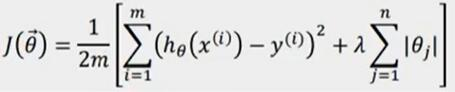• L2（Lasso回归对应平方）: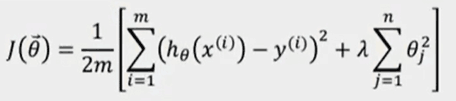• Elastic Net（L1和L2的结合）：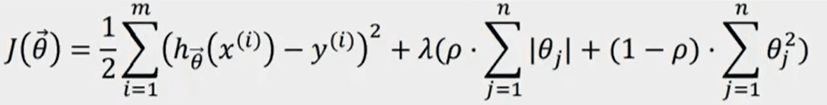结合着实际案例进行正则惩罚的使用，数据集来自于kaagle网站，数据获取网址：https://www.kaggle.com/anthonypino/melbourne-housing-market。

### 3.2.1 模块加载与数据读入

新建一个python3文件，命名为正则惩罚模型.ipynb。数据集中的字段在之前的短租房和房价预测案例分析中基本上都有介绍，然后直接导入模块进行数据读取。

import numpy as np
import matplotlib.pyplot as plt
import pandas as pd
import seaborn as sns

import warnings
warnings.filterwarnings('ignore')

dataset


输出结果如下。（数据集中共有34857条数据，21个字段）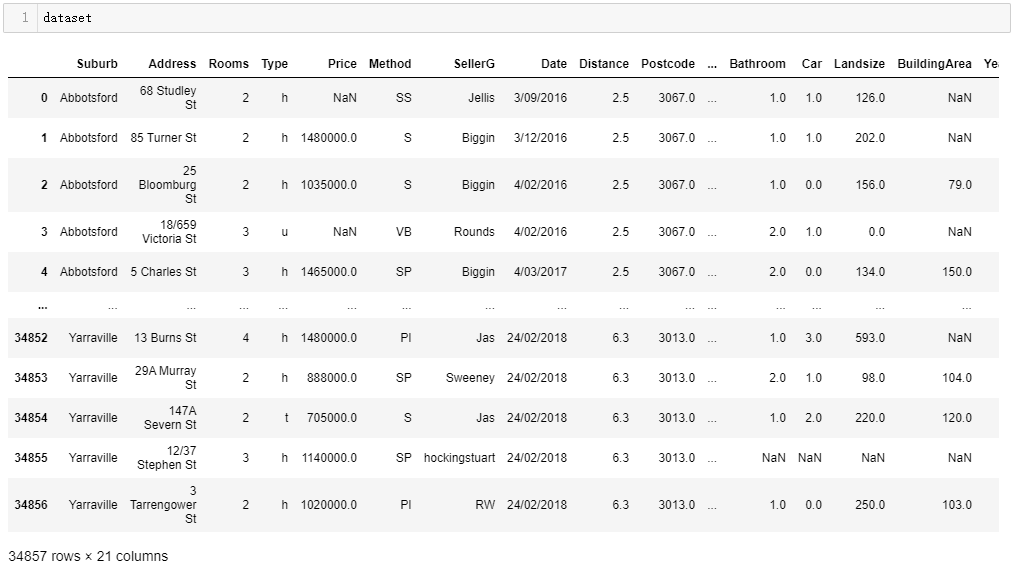通过nunique()方法可以快速查看各个字段中的唯一值的数量，用来确定一些分类字段（唯一值较少）和连续字段（唯一值较多）。输出结果如下，其中显然统计数值低于20的字段都可以认定为分类字段，比如房子所在的地区Regionname，而数据超过100的也基本上为数值连续字段。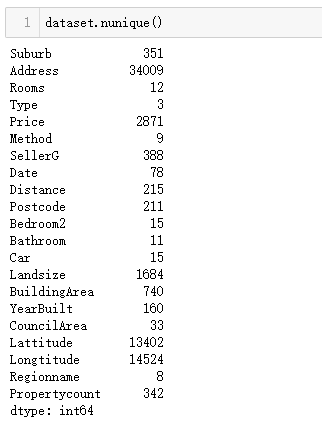### 3.2.2 特征工程

假定通过数据EDA 已经知道了哪些因素是重要数据特征 ，进行数据提取（通过corr()和heatmap()方法进行关联性探究）。本案例中主要探讨的是惩罚项如何使用，对于关联性这里不用再深究，直接提取有关联的字段构建数据集。

cols_to_use = ['Suburb', 'Rooms', 'Type', 'Method', 'SellerG', 'Regionname', 'Propertycount',
'Distance', 'CouncilArea', 'Bedroom2', 'Bathroom', 'Car', 'Landsize', 'BuildingArea', 'Price']
dataset = dataset[cols_to_use]


首先查看数据集中缺失数据情况，输出结果如下。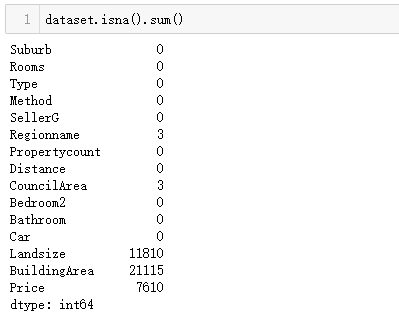然后针对与不同类型的数据进行不同方式的缺失值处理，第一类数据直接进行0填充。

cols_to_fill_zero = ['Propertycount', 'Distance', 'Bedroom2', 'Bathroom', 'Car']
dataset[cols_to_fill_zero] = dataset[cols_to_fill_zero].fillna(0)


另一种，使用数据的均值进行填充。

dataset['Landsize'] = dataset['Landsize'].fillna(dataset.Landsize.mean())
dataset['BuildingArea'] = dataset['BuildingArea'].fillna(dataset.BuildingArea.mean())


标签数据一般不进行填充，而是直接进行删除。

dataset.dropna(inplace=True)


处理完毕后，再次核实缺失值数据情况，输出结果如下。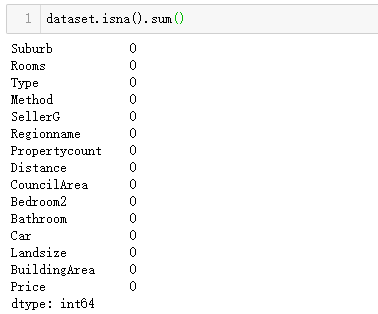前面使用nunique()方法确定部分字段属于分类数据，而对于分类数据传入数据模型之前需要进行独热编码的处理，处理的方式直接使用get_dummies()方法即可解决。由下面的输出结果可知，构建数据集中原有15个字段扩展为745个字段。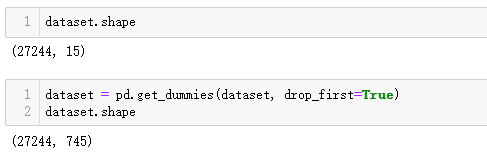至此，数据集清洗和转化的工作都完成了，下一步再次核实数据集的缺失值情况，没有缺失值后就可以进行模型的创建和应用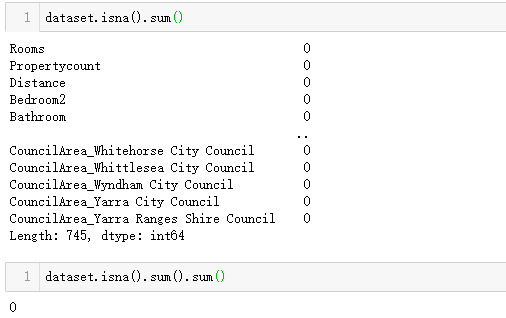### 3.2.3 模型搭建与应用

（1）构建特征数据与标签数据。

X = dataset.drop('Price', axis=1)
y = dataset['Price']


（2）划分训练集和测试集。按照9:1的比例进行拆分。

from sklearn.model_selection import train_test_split
train_X, test_X, train_y, test_y = train_test_split(X, y, test_size=0.1, random_state=2)


（3）模型初始化与训练。

from sklearn.linear_model import LinearRegression
reg = LinearRegression().fit(train_X, train_y)


（4）模型评估。

模型在训练集和测试集中的表现的结果输出如下。（很显然和上一个案例创建的模型类似，都是在训练数据上表现的还算可以，但是在测试数据上面得分小于0，存在着过拟合现象）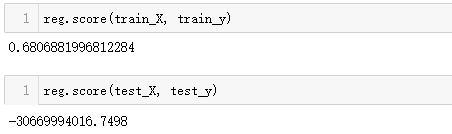（5）L1惩罚模型。

导入linear_model模块中的Lasso模型，其中的三个参数，可以调用使用手册查看对应的说明文档，本案例的重点不是对于参数的讲解，而是如何进行正则惩罚的使用，模型创建和训练的代码如下。

from sklearn import linear_model
lasso_reg = linear_model.Lasso(alpha=50, max_iter=100, tol=0.1)
lasso_reg.fit(train_X, train_y)


进一步评估模型在训练集和测试集中的得分，结果如下。（经过L1正则惩罚的模型在训练集和测试集上表现均可，且两者的得分情况相近，解决了过拟合的问题）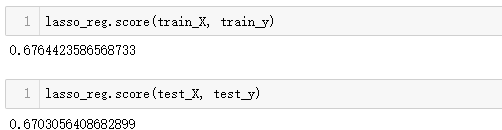（6）L2惩罚模型

使用的方式和L1类似，导入linear_model模块下的Ridge模型，三个参数与L1中的保持一致，创建和训练模型的代码如下。

from sklearn.linear_model import Ridge
ridge_reg= Ridge(alpha=50, max_iter=100, tol=0.1)
ridge_reg.fit(train_X, train_y)


进一步评估模型在训练集和测试集中的得分，结果如下。（经过L2正则惩罚的模型在训练集和测试集上表现均可，且两者的得分情况相近，解决了过拟合的问题。与L1不同的是，L2模型在测试集上的表现要比训练集好，而L1模型却相反）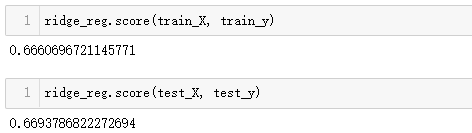关于惩罚模型的通俗的理解，以赛车比赛为例：第一名的赛车手要比第二名、第三名快7s，这个概念相当于成绩绝对碾压。而在平时的比赛中，前三名的比分咬的很死，基本上只会存在0.1s，0.2s的差距，7s这个时间差就相当于是老手和新手的之间的对比。为了提高比赛的观赏性，避免出现这种绝对优势的情景，可以在第一名的赛车上增加负重，比如增加100kg配重，此时第一名还是领先3s，那么再加配重到200kg，比赛结果中发现此时的赛车手落后原来的第二名0.3s，这次的调整就解决了比赛选手之间的实力悬殊的问题，保证了观赏性。这个过程就类似惩罚模型的工作原理，辅助对于惩罚模型的理解。

补充（经验之谈）：在处理离散数据比较多时后使用L2惩罚模型比较好，处理连续数据模型较多时候使用L1惩罚模型比较好。

展开全文回归 机器学习
• Ridge和Lasso回归代码实现–Tensorflow部分–潘登同学的机器学习笔记 python版本–3.6 ; Tensorflow版本–1.15.0 ;编辑器–Pycharm 文章目录Ridge和Lasso回归代码实现--Tensorflow部分--潘登同学的机器学习笔记Ridge...

## Ridge和Lasso回归代码实现–Tensorflow部分–潘登同学的机器学习笔记

python版本–3.6 ; Tensorflow版本–1.15.0 ;编辑器–Pycharm

# Ridge回归

• 任务:

以iris数据集的除Sepal length的数据作为X, 以Sepal length作为Y, 用X去拟合Y；

前面已经很详细介绍过了, 这里直接看代码

## 代码

import matplotlib.pyplot as plt  # 画图库
import tensorflow as tf
import numpy as np  #矩阵库
from sklearn import datasets   #导入数据集
from tensorflow.python.framework import ops   #Loss函数优化器
ops.get_default_graph()
sess = tf.Session()

X = np.array([x[1:4] for x in iris.data])
Y = np.array([y for y in iris.data])

# 声明学习率, 批量大小, 占位符和模型变量
seed = 42
tf.set_random_seed(seed)
learning_rate = 0.01
batch_size = 25
x_data = tf.placeholder(shape=[None, 3], dtype=tf.float32)
y_target = tf.placeholder(shape=[None, 1], dtype=tf.float32)
W = tf.Variable(tf.random_normal(shape=[3, 1]))
b = tf.Variable(tf.random_normal(shape=[1, 1]))

# 增加线性模型

# 声明损失函数 MSE+L2, 其为批量损失的平均值, 初始化变量, 声明优化器
L2_regularization_param = tf.reduce_mean(tf.square(W))  # L2正则项
loss = tf.expand_dims(tf.add(tf.reduce_mean(tf.square(y_target - model_output)), L2_regularization_param), 0) # MSE+L2
# tf.square 表示向量的平方  tf.reduce_mean 表示计算张量在指定维度上的平均值, 如果没有指定就是普通的算平均值
init = tf.global_variables_initializer()  # 表示初始化操作
train_step = my_opt.minimize(loss)  # 最小化Loss损失
sess.run(init)  # 运行初始化操作

# 迭代遍历, 并在随机选择的数据上进行模型训练， 迭代100次 每25次迭代输出变量值和损失值, 将其用于之后的可视化
loss_vec = []  # 储存loss随迭代次数的变化, 等一下用于画图
for i in range(100):
rand_index = np.random.choice(len(X), size=batch_size)  # 随机挑选X, 用于小批量随机梯度下降
rand_x = X[rand_index]
rand_y = np.transpose([Y[rand_index]])
# 目标：最优化损失
sess.run(train_step, feed_dict={x_data:rand_x,
y_target:rand_y})
# 更新loss值
temp_loss = sess.run(loss, feed_dict={x_data:rand_x,
y_target:rand_y})
loss_vec.append(temp_loss)
# 每25次打印
if (i+1) % 25 == 0:
print('Step:', i+1, 'w为:', sess.run(W), 'b为:', sess.run(b))
print('Loss为:', temp_loss)

# 抽取系数, 创建最佳拟合直线
slope = sess.run(W)
y_intercept = sess.run(b)
best_fit = []
for i in X:
best_fit.append(slope * i + y_intercept)
# 绘制两幅图 第一个是拟合直线(高维空间的画图就不画了) 另一个是迭代100次的L2正则损失
plt.figure(2)
plt.plot(loss_vec, 'k--')
plt.title('Ridge loss per Generation')
plt.xlabel('Generation')
plt.ylabel('loss')
plt.show()


## 结果# Lasso回归

• 任务:

以iris数据集的除Sepal length的数据作为X, 以Sepal length作为Y, 用X去拟合Y；

## 代码

import matplotlib.pyplot as plt  # 画图库
import tensorflow as tf
import numpy as np  #矩阵库
from sklearn import datasets   #导入数据集
from tensorflow.python.framework import ops   #Loss函数优化器
ops.get_default_graph()
sess = tf.Session()

X = np.array([x[1:4] for x in iris.data])
Y = np.array([y for y in iris.data])

# 声明学习率, 批量大小, 占位符和模型变量
seed = 42
tf.set_random_seed(seed)
learning_rate = 0.01
batch_size = 25
x_data = tf.placeholder(shape=[None, 3], dtype=tf.float32)
y_target = tf.placeholder(shape=[None, 1], dtype=tf.float32)
W = tf.Variable(tf.random_normal(shape=[3, 1]))
b = tf.Variable(tf.random_normal(shape=[1, 1]))

# 增加线性模型

# 声明损失函数 MSE+L2, 其为批量损失的平均值, 初始化变量, 声明优化器
L1_regularization_param = tf.reduce_mean(tf.abs(W))  # L1正则项
loss = tf.expand_dims(tf.add(tf.reduce_mean(tf.square(y_target - model_output)), L1_regularization_param), 0) # MSE+L1
# tf.square 表示向量的平方  tf.reduce_mean 表示计算张量在指定维度上的平均值, 如果没有指定就是普通的算平均值
init = tf.global_variables_initializer()  # 表示初始化操作
train_step = my_opt.minimize(loss)  # 最小化Loss损失
sess.run(init)  # 运行初始化操作

# 迭代遍历, 并在随机选择的数据上进行模型训练， 迭代100次 每25次迭代输出变量值和损失值, 将其用于之后的可视化
loss_vec = []  # 储存loss随迭代次数的变化, 等一下用于画图
for i in range(100):
rand_index = np.random.choice(len(X), size=batch_size)  # 随机挑选X, 用于小批量随机梯度下降
rand_x = X[rand_index]
rand_y = np.transpose([Y[rand_index]])
# 目标：最优化损失
sess.run(train_step, feed_dict={x_data:rand_x,
y_target:rand_y})
# 更新loss值
temp_loss = sess.run(loss, feed_dict={x_data:rand_x,
y_target:rand_y})
loss_vec.append(temp_loss)
# 每25次打印
if (i+1) % 25 == 0:
print('Step:', i+1, 'w为:', sess.run(W), 'b为:', sess.run(b))
print('Loss为:', temp_loss)

# 抽取系数, 创建最佳拟合直线
slope = sess.run(W)
y_intercept = sess.run(b)
best_fit = []
for i in X:
best_fit.append(slope * i + y_intercept)
# 绘制两幅图 第一个是拟合直线(高维空间的画图就不画了) 另一个是迭代100次的L2正则损失
plt.figure(2)
plt.plot(loss_vec, 'k--')
plt.title('Lasso loss per Generation')
plt.xlabel('Generation')
plt.ylabel('loss')
plt.show()


## 结果# ElasticNet

还记得吗？ ElasticNet是即用L1也用L2

• 任务:

以iris数据集的除Sepal length的数据作为X, 以Sepal length作为Y, 用X去拟合Y；

## 代码

import matplotlib.pyplot as plt  # 画图库
import tensorflow as tf
import numpy as np  #矩阵库
from sklearn import datasets   #导入数据集
from tensorflow.python.framework import ops   #Loss函数优化器
ops.get_default_graph()
sess = tf.Session()

X = np.array([x[1:4] for x in iris.data])
Y = np.array([y for y in iris.data])

# 声明学习率, 批量大小, 占位符和模型变量
seed = 42
tf.set_random_seed(seed)
learning_rate = 0.01
batch_size = 25
x_data = tf.placeholder(shape=[None, 3], dtype=tf.float32)
y_target = tf.placeholder(shape=[None, 1], dtype=tf.float32)
W = tf.Variable(tf.random_normal(shape=[3, 1]))
b = tf.Variable(tf.random_normal(shape=[1, 1]))

# 增加线性模型

# 声明损失函数 MSE+L2, 其为批量损失的平均值, 初始化变量, 声明优化器
L1_regularization_param = tf.reduce_mean(tf.abs(W))  # L1正则项
L2_regularization_param = tf.reduce_mean(tf.square(W))  # L2正则项
regularization_param = tf.add(L1_regularization_param, L2_regularization_param) # L1 + L2
loss = tf.expand_dims(tf.add(tf.reduce_mean(tf.square(y_target - model_output)), regularization_param), 0) # MSE+ L1 + L2
# tf.square 表示向量的平方  tf.reduce_mean 表示计算张量在指定维度上的平均值, 如果没有指定就是普通的算平均值
init = tf.global_variables_initializer()  # 表示初始化操作
train_step = my_opt.minimize(loss)  # 最小化Loss损失
sess.run(init)  # 运行初始化操作

# 迭代遍历, 并在随机选择的数据上进行模型训练， 迭代100次 每25次迭代输出变量值和损失值, 将其用于之后的可视化
loss_vec = []  # 储存loss随迭代次数的变化, 等一下用于画图
for i in range(100):
rand_index = np.random.choice(len(X), size=batch_size)  # 随机挑选X, 用于小批量随机梯度下降
rand_x = X[rand_index]
rand_y = np.transpose([Y[rand_index]])
# 目标：最优化损失
sess.run(train_step, feed_dict={x_data:rand_x,
y_target:rand_y})
# 更新loss值
temp_loss = sess.run(loss, feed_dict={x_data:rand_x,
y_target:rand_y})
loss_vec.append(temp_loss)
# 每25次打印
if (i+1) % 25 == 0:
print('Step:', i+1, 'w为:', sess.run(W), 'b为:', sess.run(b))
print('Loss为:', temp_loss)

# 抽取系数, 创建最佳拟合直线
slope = sess.run(W)
y_intercept = sess.run(b)
best_fit = []
for i in X:
best_fit.append(slope * i + y_intercept)
# 绘制两幅图 第一个是拟合直线(高维空间的画图就不画了) 另一个是迭代100次的L2正则损失
plt.figure(2)
plt.plot(loss_vec, 'k--')
plt.title('ElasticNet loss per Generation')
plt.xlabel('Generation')
plt.ylabel('loss')
plt.show()


## 结果Ridge和Lasso回归代码实现–Tensorflow部分就是这样了, 继续下一章吧！pd的Machine Learning

展开全文tensorflow 回归 python
• ## Ridge和Lasso回归

千次阅读 2021-02-08 03:16:20
上周看了看回归方面的知识，顺便复（xue）习一下Ridge（岭回归）和Lasso回归（套索回归）。瞅到了一篇英文博客讲得不错，翻译一下 本文翻译自 Ridge and Lasso Regression 本文是一篇Josh Starmer关于 视频的总结...回归 正则化
• 在这篇文章中，我们将首先看看Lasso和Ridge回归中一些常见的错误，然后我将描述我通常采取的步骤来优化超参数。代码是用Python编写的，我们主要依赖scikit-learn。本文章主要关注Lasso的例子，但其基本理论与Ridge...机器学习 数据挖掘 线性回归 lasso回归
• 手写算法-python代码实现Ridge回归Ridge简介Ridge回归分析与python代码实现1、标准方程法实现Ridge回归2、梯度下降法实现Ridge回归调用sklearn对比 Ridge简介 前面2篇文章，我们介绍了过拟合与正则化，比较全面的讲...python 机器学习 算法 正则化
• 在这篇文章中，我们将首先看看Lasso和Ridge回归中一些常见的错误，然后我将描述我通常采取的步骤来优化超参数。代码是用Python编写的，我们主要依赖scikit-learn。本文章主...python 机器学习 人工智能 深度学习 java
• 机器学习算法系列（四）- 岭回归算法（Ridge Regression Algorithm）岭回归 回归算法
• 目录1 基本库导入2 线性回归2.1 线性模型性能2.2 使用更高维的数据集3 岭回归-Ridge3.1 Ridge原理及应用3.2 Ridge调参3.3 为什么要用Ridge4 Lasso4.1 基本原理及应用4.2 Lasso调参4.3 为什么要用Lasso4.4 Lasso和...python 机器学习 算法
• 描述为什么在Ridge和Lasso回归之前必须进行标准化 比较和对比套索，岭和非正规回归 在scikit-learn中使用Lasso和Ridge回归 我们的回归成本函数 从先前的课程中，您知道在求解线性回归时，可以将成本函数表示为  \ ...
• 文章目录1.形式化定义2.梯度下降法1)举例2）数学原理3）代码演示3.梯度下降法求解线性回归1）理论2）线性回归代码实现梯度下降算法4....使用sklearn实现线性回归、Ridge、 LASSO 、ElasyicNet1）Scik机器学习 算法 python
• ## 5.3 Ridge 回归分析

千次阅读 2020-07-24 11:24:13
岭回归(英文名：ridge regression, Tikhonov regularization)是一种专用于共线性数据分析的有偏估计回归方法，实质上是一种改良的最小二乘估计法，通过放弃最小二乘法的无偏性，以损失部分信息、降低精度为代价获得......